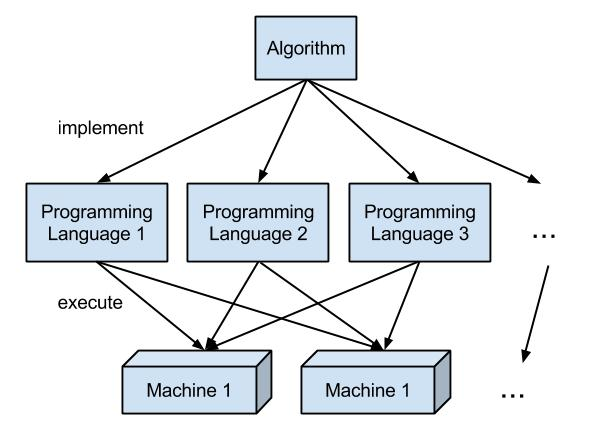# What is the Difference Between Backtracking and Branch and Bound

The main difference between backtracking and branch and bound is that the backtracking is an algorithm for capturing some or all solutions to given computational issues, especially for constraint satisfaction issues while branch and bound is an algorithm to find the optimal solution to many optimization problems, especially in discrete and combinatorial optimization.

An algorithm is a methodical sequence of steps to solve a particular problem. There are various algorithms, and two of them are backtracking and branch and bound.

### Key Areas Covered

1. What is Backtracking
– Definition, Functionality
2. What is Branch and Bound
– Definition, Functionality
3. What is the Difference Between Backtracking and Branch and Bound
– Comparison of Key Differences

### Key Terms

Backtracking, Branch and Bound## What is Backtracking

Backtracking is an algorithm that solves the problem in a recursive manner. It is a systematic way of trying different sequences of decisions to find the correct decision. It figures out the solution by searching the solution space of the given problem methodically.All solutions for backtracking need to satisfy a complex set of explicit and implicit constraints. The explicit constraint limits every vector element to be selected from the given set. Moreover, implicit constraint finds the tuples in the solution space that can satisfy the criterion function.

## What is Branch and Bound

Branch and bound is more suitable for situations where we cannot apply the greedy method and dynamic programming. Usually, this algorithm is slow as it requires exponential time complexities during the worst case, but sometimes it works with reasonable efficiency. However, this method helps to determine global optimization in non-convex problems.

Further, to solve a problem, this method divides the given subproblem into at least two new restricted subproblems.

## Difference Between Backtracking and Branch and Bound

### Definition

Backtracking is an algorithm for finding all solutions to some computational problems, notably constraint satisfaction problems that incrementally builds candidates to the solutions. Branch and bound is an algorithm for discrete and combinatorial optimization problems and mathematical optimization. Thus, this is the main difference between backtracking and branch and bound.

### Process

Furthermore, backtracking finds the solution to the overall issue by finding a solution to the first subproblem and them recursively solving other subproblems based on the solution of the first issue. However, branch and bound solves a given problem by dividing it into at least two new restricted subproblems. Hence, this is another difference between backtracking and branch and bound.

### Efficiency

Moreover, efficiency is a major difference between backtracking and branch and bound. Backtracking is more efficient than the Branch and Bound algorithm.

### Conclusion

Backtracking is an algorithm for capturing some or all solutions to given computational issues, especially for constraint satisfaction issues. Branch and Bound, on the other hand, is an algorithm to find optimal solutions to many optimization problems, especially in discrete and combinatorial optimization. That is the main difference between Backtracking and Branch and Bound.

##### Reference:

1. “DAA Algorithm Design Techniques – Javatpoint.” Www.javatpoint.com, Available here.
2. “Backtracking Introduction – Javatpoint.” Www.javatpoint.com, Available here.
3. “Backtracking.” Wikipedia, Wikimedia Foundation, 7 Dec. 2018, Available here.
4. “Branch and Bound.” Wikipedia, Wikimedia Foundation, 8 Oct. 2018, Available here.
5. “What Is Backtracking? – Definition from Techopedia.” Techopedia.com, Available here.

##### Image Courtesy:

1. “Algorithms v.s. Programming Languages” By Lubaochuan – Own work (CC BY-SA 4.0) via Commons Wikimedia